# Schubert polynomials

(diff) ← Older revision | Latest revision (diff) | Newer revision → (diff)

Polynomials introduced by A. Lascoux and M.-P. Schützenberger [a18] as distinguished polynomial representatives of Schubert cycles (cf. also Schubert cycle) in the cohomology ring of the manifold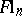of complete flags in. This extended work by I.N. Bernshtein, I.M. Gel'fand and S.I. Gel'fand [a1] and M. Demazure [a8], who gave algorithms for computing representatives of Schubert cycles in the co-invariant algebra, which is isomorphic to the cohomology ring of[a6]: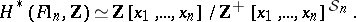Here,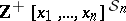is the ideal generated by the non-constant polynomials that are symmetric in. See [a19] for an elegant algebraic treatment of Schubert polynomials, and [a13] and [a20] for a more geometric treatment.

For each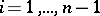, let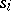be the transposition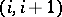in the symmetric group, which acts on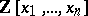. The divided difference operator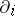is defined by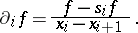These satisfy(a1)

If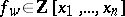is a representative of the Schubert cycle, then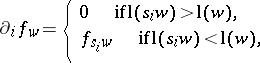whereis the length of a permutation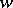and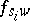represents the Schubert cycle. Given a fixed polynomial representative of the Schubert cycle(the class of a point as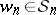is the longest element), successive application of divided difference operators gives polynomial representatives of all Schubert cycles, which are independent of the choices involved, by (a1).

The choice of the representative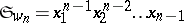forgives the Schubert polynomials. Since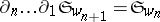, Schubert polynomials are independent ofand give polynomials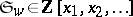for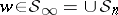. These form a basis for this polynomial ring, and every Schur polynomial is also a Schubert polynomial.

The transition formula gives another recursive construction of Schubert polynomials. For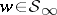, letbe the last descent of(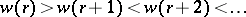) and defineby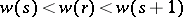. Set, where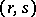is the transposition. Thenthe sum over all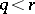with. This formula gives an algorithm to compute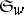as the permutations that appear on the right-hand side are either shorter thanor precede it in reverse lexicographic order, and the minimal such permutationof lengthhas.

The transition formula shows that the Schubert polynomialis a sum of monomials with non-negative integral coefficients. There are several explicit formulas for the coefficient of a monomial in a Schubert polynomial, either in terms of the weak order of the symmetric group [a3], [a5], [a12], an intersection number [a15] or the Bruhat order [a4]. An elegant conjectural formula of A. Kohnert [a16] remains unproven (as of 2000). The Schubert polynomialforis also the normal form reduction of any polynomial representative of the Schubert cycle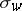with respect to the degree-reverse lexicographic term order onwith.

The above-mentioned results of [a6], [a1], [a8] are valid more generally for any flag manifoldwitha semi-simple reductive group anda Borel subgroup. Whenis an orthogonal or symplectic group, there are competing theories of Schubert polynomials [a2], [a10], [a17], each with own merits. There are also double Schubert polynomials suited for computations of degeneracy loci [a11], quantum Schubert polynomials [a9], [a7] and universal Schubert polynomials [a14].

How to Cite This Entry:
Schubert polynomials. Encyclopedia of Mathematics. URL: http://encyclopediaofmath.org/index.php?title=Schubert_polynomials&oldid=14636
This article was adapted from an original article by Frank Sottile (originator), which appeared in Encyclopedia of Mathematics - ISBN 1402006098. See original article